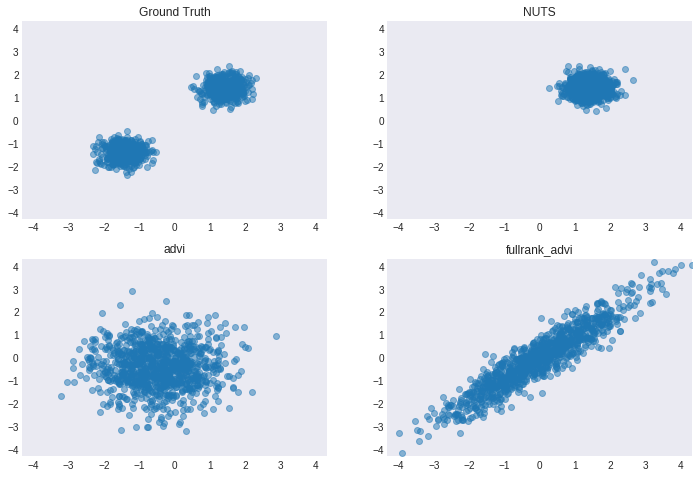In :
import theano
theano.config.compute_test_value = 'raise'  # funny bug when first making a theano variable


A few computational experiments with ADVI, comparing NUTS, mean-field ADVI, and full-rank ADVI approximations of a distribution.

In :
%matplotlib inline

import matplotlib
import numpy as np
import pymc3 as pm
import matplotlib.pyplot as plt
import theano.tensor as tt

plt.style.use('seaborn-dark')

/home/colin/miniconda3/envs/scratch3.6/lib/python3.6/site-packages/h5py/__init__.py:36: FutureWarning: Conversion of the second argument of issubdtype from float to np.floating is deprecated. In future, it will be treated as np.float64 == np.dtype(float).type.
from ._conv import register_converters as _register_converters

In :
def _get_bounds(samples):
"""Helper function to draw consistent bounds"""
x_max, y_max = 0, 0
for x_draws, y_draws in samples.values():
x_max = max(x_max, np.abs(x_draws).max())
y_max = max(y_max, np.abs(y_draws).max())
top = max(x_max, y_max)
return (-top, top)

def density_model(Ω):
"""This is the "modeling" step.

We are using PyMC3, and assuming we get an object Ω that represents some joint
distribution. We require Ω to have two methods: logp and random. The logp should also
be defined in a Theano-compatible fashion so it can compute the gradients.
"""
with pm.Model() as model:
omega = pm.DensityDist('Ω', Ω.logp, shape=2)
return model

def sampler(Ω, N=1000):
"""This is the "inference" step

Note that the only added difficulty in using ADVI is that we have a call to pm.fit in order to learn
the parameters for the mean-field or full-rank approximation.
"""
samples = {'Ground Truth': Ω.random(size=N).T}
with density_model(Ω):
samples['NUTS'] = pm.sample(N, step=pm.NUTS(), chains=1)['Ω'].T

inference = pm.fit(n=30000, method=method)
samples[method] = inference.sample(N)['Ω'].T

return samples

def plotter(samples):
"""Helper to plot the output of sampler.  A little flexible in case we want to add more VI methods.
"""
size = int(np.ceil(len(samples) ** 0.5))
fix, axs = plt.subplots(size, size, figsize=(12, 8))
bounds = _get_bounds(samples)
for (label, (x, y)), ax in zip(samples.items(), axs.ravel()):
ax.plot(x, y, 'o', alpha = 0.5)
ax.set_title(label)
ax.axes.set_xlim(bounds)
ax.axes.set_ylim(bounds)

def sample_and_plot(dist):
"""For the lazy"""
samples = sampler(dist)
plotter(samples)


# 1. Ill-conditioned Gaussian¶

In :
mu = tt.zeros(2,)
cov = tt.as_tensor([[1e-2, 0.], [0., 1e2]])
Ω = pm.MvNormal.dist(mu=mu, cov=cov, shape=2, testval=mu)

In :
sample_and_plot(Ω)

/home/colin/miniconda3/envs/scratch3.6/lib/python3.6/site-packages/pymc3/model.py:384: FutureWarning: Conversion of the second argument of issubdtype from float to np.floating is deprecated. In future, it will be treated as np.float64 == np.dtype(float).type.
if not np.issubdtype(var.dtype, float):
Sequential sampling (1 chains in 1 job)
NUTS: [Ω]
100%|██████████| 1500/1500 [00:02<00:00, 711.69it/s]
Only one chain was sampled, this makes it impossible to run some convergence checks
Average Loss = 0.09657: 100%|██████████| 30000/30000 [00:10<00:00, 2821.92it/s]
Finished [100%]: Average Loss = 0.097131
Average Loss = 0.063651: 100%|██████████| 30000/30000 [00:14<00:00, 2015.99it/s]
Finished [100%]: Average Loss = 0.06198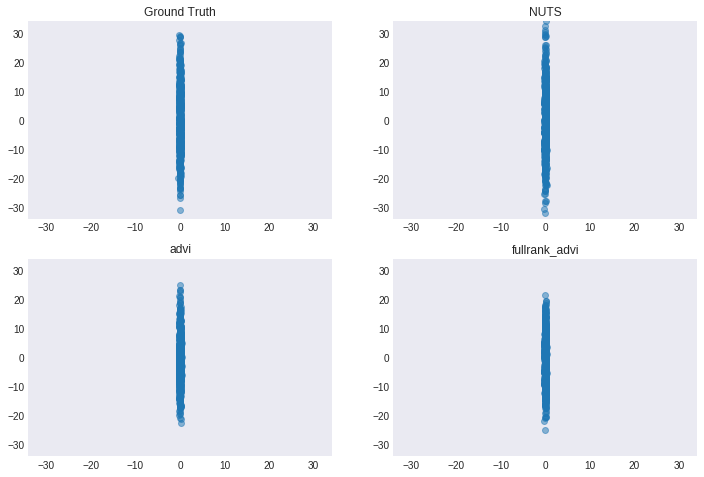# 2. Strongly correlated Gaussian¶

In :
mu = tt.zeros(2,)
cov = tt.as_tensor([[50.05, -49.95], [-49.95, 50.05]])
Ω = pm.MvNormal.dist(mu=mu, cov=cov, shape=2, testval=mu)

In :
sample_and_plot(Ω)

/home/colin/miniconda3/envs/scratch3.6/lib/python3.6/site-packages/pymc3/model.py:384: FutureWarning: Conversion of the second argument of issubdtype from float to np.floating is deprecated. In future, it will be treated as np.float64 == np.dtype(float).type.
if not np.issubdtype(var.dtype, float):
Sequential sampling (1 chains in 1 job)
NUTS: [Ω]
100%|██████████| 1500/1500 [00:06<00:00, 215.85it/s]
Only one chain was sampled, this makes it impossible to run some convergence checks
Average Loss = 2.7394: 100%|██████████| 30000/30000 [00:09<00:00, 3022.32it/s]
Finished [100%]: Average Loss = 2.744
Average Loss = 0.83846: 100%|██████████| 30000/30000 [00:17<00:00, 1712.92it/s]
Finished [100%]: Average Loss = 0.83957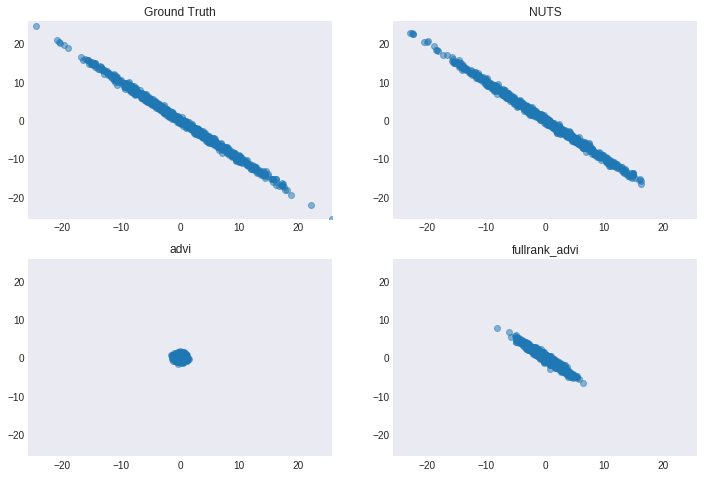# 3. Mixture of Gaussians¶

In :
class MoG(object):
def __init__(self, centers, sds):
mu_1, mu_2 = centers
cov_1 = tt.as_tensor([[sds, 0], [0, sds]])
cov_2 = tt.as_tensor([[sds, 0], [0, sds]])

self.rvs = [pm.MvNormal.dist(mu=mu_1, cov=cov_1, shape=2),
pm.MvNormal.dist(mu=mu_2, cov=cov_2, shape=2)]

def random(self, size=1):
return np.array([rv.random() for rv in np.random.choice(self.rvs, size=size)])

def logp(self, value):
return pm.math.logsumexp([rv.logp(value) for rv in self.rvs]) - np.log(len(self.rvs))

In :
Ω = MoG(centers=[np.array([-2, 0]), np.array([2, 0])], sds=[0.1, 0.1])

In :
sample_and_plot(Ω)

/home/colin/miniconda3/envs/scratch3.6/lib/python3.6/site-packages/pymc3/model.py:384: FutureWarning: Conversion of the second argument of issubdtype from float to np.floating is deprecated. In future, it will be treated as np.float64 == np.dtype(float).type.
if not np.issubdtype(var.dtype, float):
Sequential sampling (1 chains in 1 job)
NUTS: [Ω]
100%|██████████| 1500/1500 [00:01<00:00, 957.48it/s]
Only one chain was sampled, this makes it impossible to run some convergence checks
Average Loss = 5.969: 100%|██████████| 30000/30000 [00:14<00:00, 2121.74it/s]
Finished [100%]: Average Loss = 5.9217
Average Loss = 6.3004: 100%|██████████| 30000/30000 [00:21<00:00, 1409.53it/s]
Finished [100%]: Average Loss = 6.2906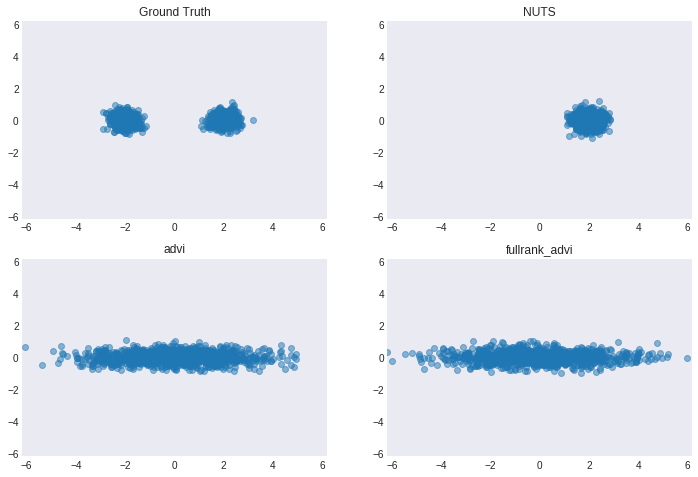# 4. Mixture of Gaussians with different scales¶

In :
Ω = MoG(centers=[np.array([-4, 0]), np.array([4, 0])], sds=[2, 0.1])

In :
sample_and_plot(Ω)

/home/colin/miniconda3/envs/scratch3.6/lib/python3.6/site-packages/pymc3/model.py:384: FutureWarning: Conversion of the second argument of issubdtype from float to np.floating is deprecated. In future, it will be treated as np.float64 == np.dtype(float).type.
if not np.issubdtype(var.dtype, float):
Sequential sampling (1 chains in 1 job)
NUTS: [Ω]
100%|██████████| 1500/1500 [00:01<00:00, 932.83it/s]
Only one chain was sampled, this makes it impossible to run some convergence checks
Average Loss = 0.70752: 100%|██████████| 30000/30000 [00:13<00:00, 2201.93it/s]
Finished [100%]: Average Loss = 0.70748
Average Loss = 0.71185: 100%|██████████| 30000/30000 [00:20<00:00, 1437.01it/s]
Finished [100%]: Average Loss = 0.71251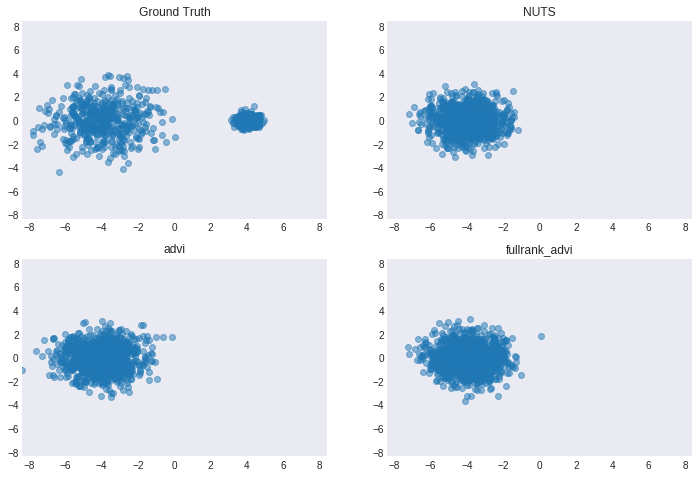# 5. Mixture of non-axis-aligned Gaussians¶

In :
θ = np.pi / 4
rot_45 = np.array([[np.cos(θ), -np.sin(θ)], [np.sin(θ), np.cos(θ)]])
Ω = MoG(centers=[rot_45.dot(np.array([-2, 0])), rot_45.dot(np.array([2, 0]))], sds=[0.1, 0.1])

In :
sample_and_plot(Ω)

/home/colin/miniconda3/envs/scratch3.6/lib/python3.6/site-packages/pymc3/model.py:384: FutureWarning: Conversion of the second argument of issubdtype from float to np.floating is deprecated. In future, it will be treated as np.float64 == np.dtype(float).type.
if not np.issubdtype(var.dtype, float):
Sequential sampling (1 chains in 1 job)
NUTS: [Ω]
100%|██████████| 1500/1500 [00:01<00:00, 936.08it/s]
Only one chain was sampled, this makes it impossible to run some convergence checks
Average Loss = 10.593: 100%|██████████| 30000/30000 [00:16<00:00, 1829.69it/s]
Finished [100%]: Average Loss = 10.65
Average Loss = 6.4672: 100%|██████████| 30000/30000 [00:20<00:00, 1463.15it/s]
Finished [100%]: Average Loss = 6.4597